High School Physics : Gas Laws

Example Questions

Example Question #1 : Understanding Isovolumetric Processes

A gas undergoes a thermodynamic process. During this process, no work is done. Which of the following is the best description of the process?

Isobatic

Isovolumetric

Isothermal

Isobaric

Isovolumetric

Explanation:

There are two equations that may be relevant for this problem: the first law of thermodynamics and the work equation.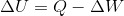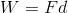If the work done is zero, then we can conclude that: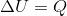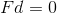So, the change in internal energy is equal to the heat input, and either the force or the distance will remain unchanged. Of these options, only an isovolumetric process is given as a possible answer. In this process, the size of the gas will remain constant, leading to a displacement of zero in the work equation.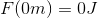Example Question #2 : Understanding Isovolumetric Processes

Which of these is an example of an isovolumetric process?

The temperature of a gas is raised, all of the energy transfers to work, and no heat is released

Work is done on a gas, its temperature rises, and no energy is released in the form of heat

A gas takes in heat, does work, and the internal temperature of the gas does not change

A gas maintains a constant level of heat, temperature, and work

The temperature of a gas is raised, all of the energy transfers to heat, and no work is done

The temperature of a gas is raised, all of the energy transfers to heat, and no work is done

Explanation:

When evaluating different types of processes, the most useful formula is usually the first law of thermodynamics: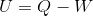In an isovolumetric process there is no change in volume, which means that the container does not expand and there is no change in distance or radius. Based on the equation for work, we can see that an isovolumetric process must also have zero work done.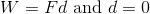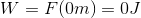Returning to the first equation, we can conclude that the change in internal energy (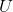) must be equal to the heat energy input (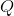).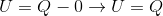Essentially, all energy that enters the system as heat is converted to internal energy because no work is done by the system. The result of this increase in internal energy is an increase in temperature.